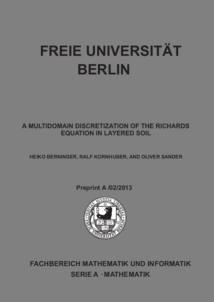Repository: Freie Universität Berlin, Math Department

# A multidomain discretization of the Richards equation in layered soil

Berninger, H. and Kornhuber, R. and Sander, O. (2015) A multidomain discretization of the Richards equation in layered soil. Computational Geosciences, 19 (1). pp. 213-232. ISSN 1420-0597Preview

1MB

Official URL: http://dx.doi.org/10.1007/s10596-014-9461-8

## Abstract

We consider the Richards equation on a domain that is decomposed into nonoverlapping layers, i.e., the decomposition has no cross points. We assume that the saturation and permeability functions are space-independent on each subdomain. Kirchhoff transformation of each subdomain problem separately then leads to a set of semilinear equations, which can each be solved efficiently using monotone multigrid. The transformed subdomain problems are coupled by nonlinear continuity and flux conditions. This nonlinear coupled problem can be solved using substructuring methods like the Dirichlet–Neumann or Robin iteration. We give several numerical examples showing the discretization error, the solver robustness under variations of the soil parameters, and a hydrological example with four soil layers and surface water.

Item Type: Article Saturated, unsaturated groundwater flow, Seepage, Heterogeneous soil, Kirchhoff transformation, Monotone multigrid, Domain decomposition, Nonlinear transmission conditions Mathematical and Computer Sciences > Mathematics > Numerical Analysis Department of Mathematics and Computer Science > Institute of Mathematics > Computational PDEs Group 1785 Ekaterina Engel 17 Feb 2016 09:19 03 Mar 2017 14:41

Repository Staff Only: item control page